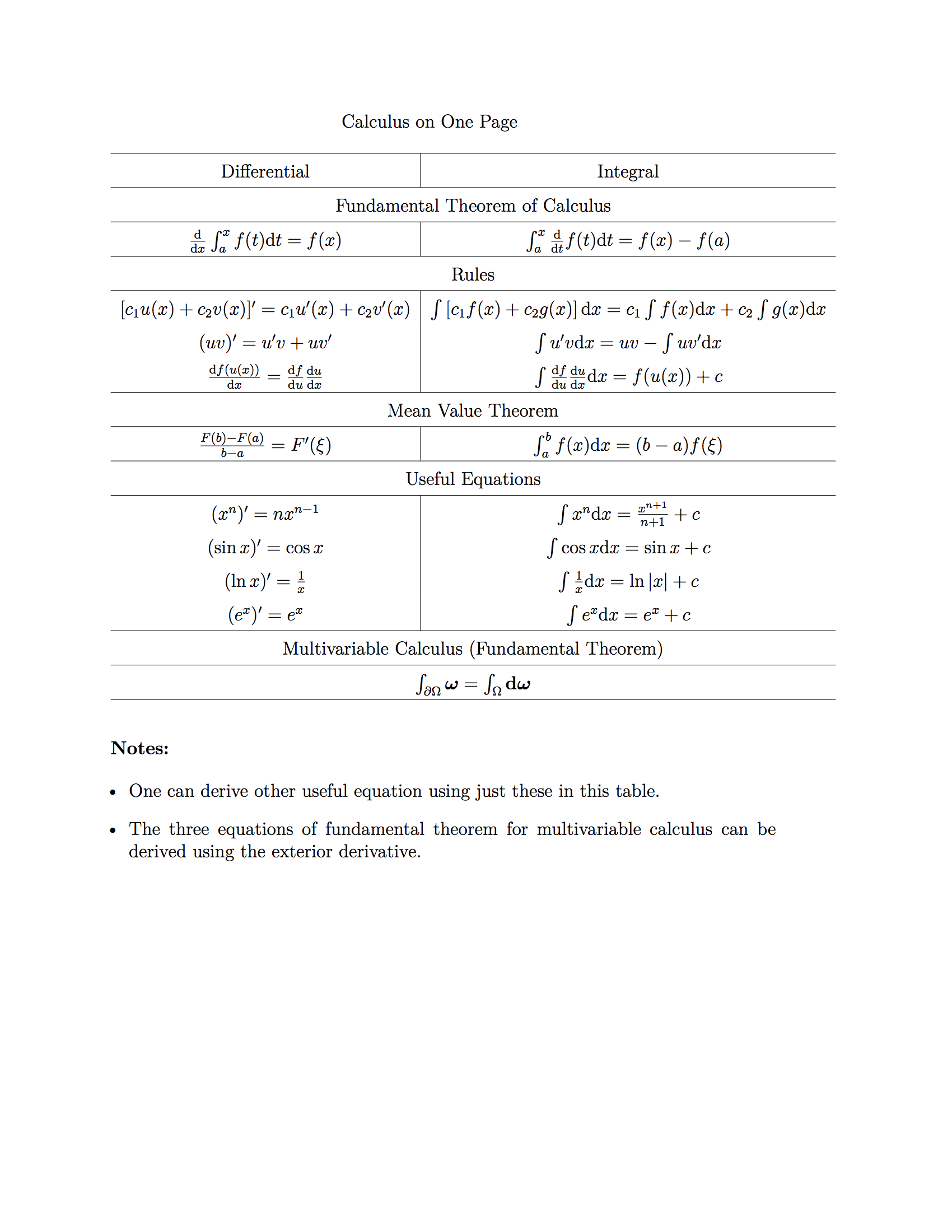# Calculus¶Fig. 11 LaTeX source of this image is here .

## Integrals¶

Sometimes a integral on Real plane can be very hard, one of the techniques is to work on Complex plane and use contour integral.

1. Contours: use Ghost Contours so that we don’t need to calculate these complicated integrals.

2. Branch Cut: cuts are needed if we have got branch points on the complex plane.

3. Residue Theorem: we can write down the integral by calculating the residue of the integrand,

$\int_C f(z) \mathrm dz = 2\pi i \sum_j \text{Residue}(f(z_j)),$

where $$z_j$$ are the poles.